Скачать презентацию 1 CALCULATIONS FOR NAF BASE MUDS 2

calculations_only_-nt.ppt

• Размер: 242 Кб
• Количество слайдов: 17

## Описание презентации 1 CALCULATIONS FOR NAF BASE MUDS 2 по слайдам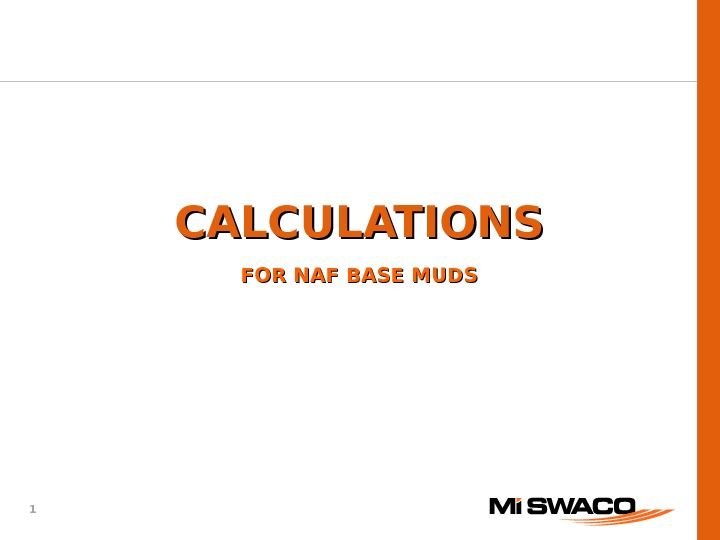1 CALCULATIONS FOR NAF BASE MUDS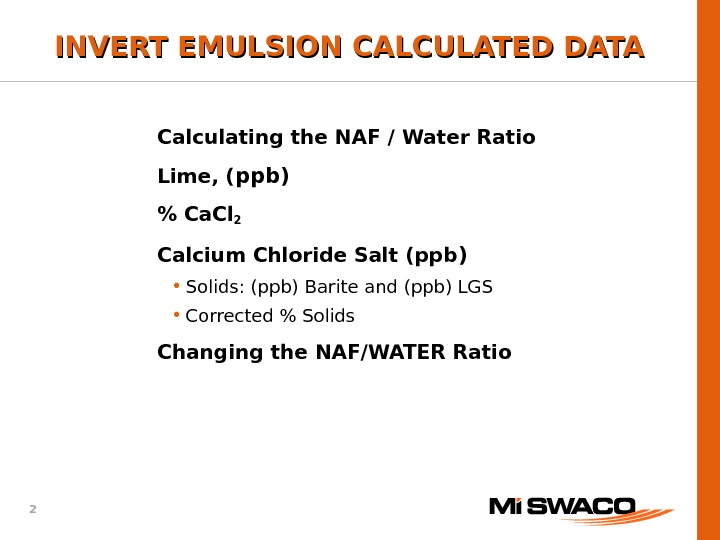2 Calculating the NAF / Water Ratio Lime, ( ppb) % Ca. Cl 2 Calcium Chloride Salt (ppb ) • Solids: (ppb) Barite and (ppb) LGS • Corrected % Solids Changing the NAF/WATER Ratio. INVERT EMULSION CALCULATED DAT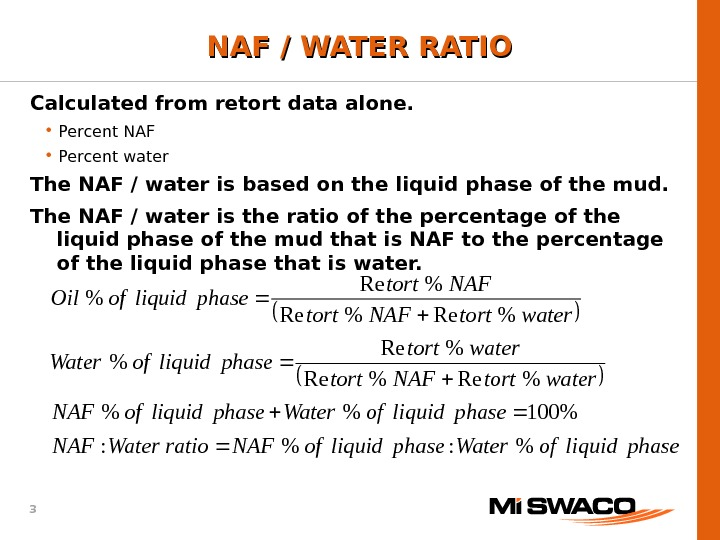3 phaseliquidof. Waterphaseliquidof. NAFratio. Water. NAF phaseliquidof. Waterphaseliquidof. NAF watertort. NAFtort watertort phaseliquidof. Water watertort. NAFtort phaseliquidof. Oil %: %: %100%% %Re%Re %Re % NAF / WATER RATIO Calculated from retort data alone. • Percent NAF • Percent water The NAF / water is based on the liquid phase of the mud. The NAF / water is the ratio of the percentage of the liquid phase of the mud that is NAF to the percentage of the liquid phase that is water.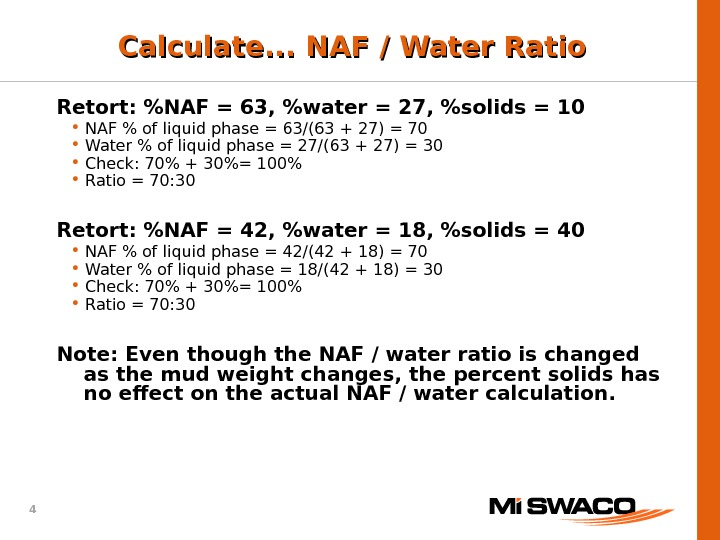4 Retort: %NAF = 63, %water = 27, %solids = 10 • NAF % of liquid phase = 63/(63 + 27) = 70 • Water % of liquid phase = 27/(63 + 27) = 30 • Check: 70% + 30%= 100% • Ratio = 70: 30 Retort: %NAF = 42, %water = 18, %solids = 40 • NAF % of liquid phase = 42/(42 + 18) = 70 • Water % of liquid phase = 18/(42 + 18) = 30 • Check: 70% + 30%= 100% • Ratio = 70: 30 Note: Even though the NAF / water ratio is changed as the mud weight changes, the percent solids has no effect on the actual NAF / water calculation. Calculate. . . NAF / Water Ratio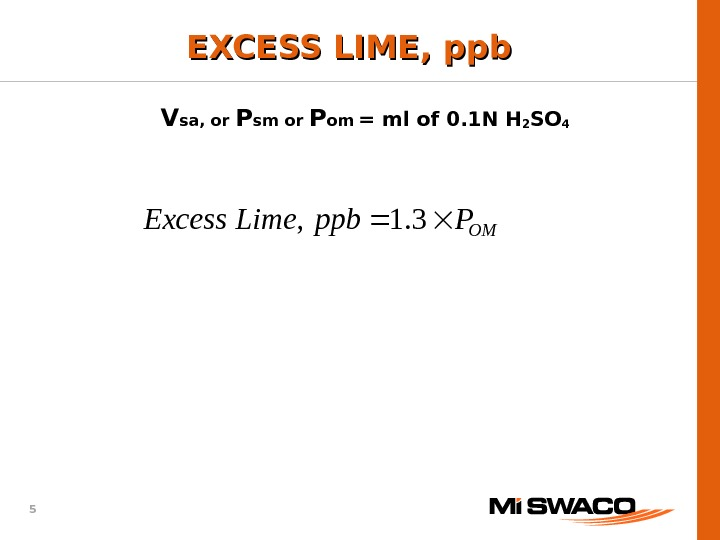5 OMPppb. Lime. Excess 3. 1, EXCESS LIME, ppb V sa, or P sm or P om = ml of 0. 1 N H 2 SO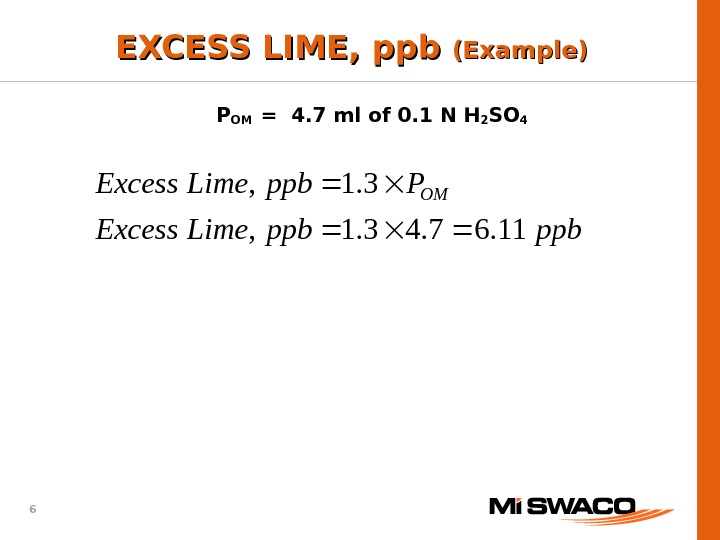6 ppbppb. Lime. Excess Pppb. Lime. Excess OM 11. 67. 43. 1, EXCESS LIME, ppb (Example) P OM = 4. 7 ml of 0. 1 N H 2 SO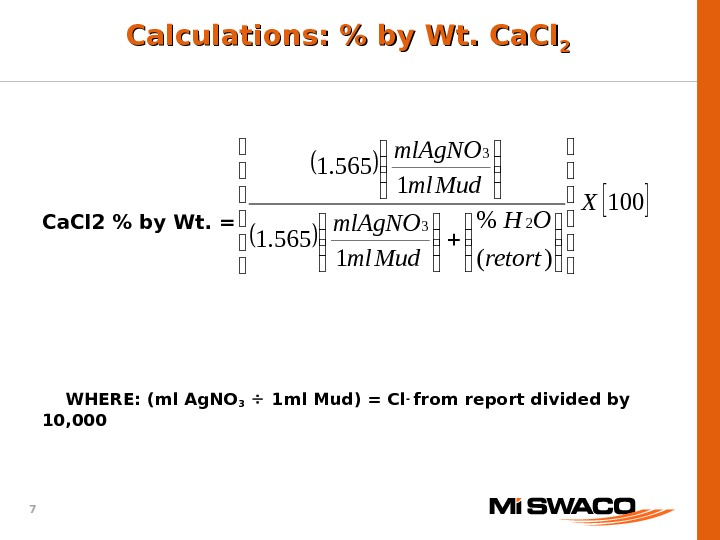7 Calculations: % by Wt. Ca. Cl 22 Ca. Cl 2 % by Wt. = WHERE: (ml Ag. NO 3 1 ml Mud) = Cl — from report divided by 10, 000 100 )(% 1565. 1 2 3 3 X retort OH Mudmlml. Ag. NO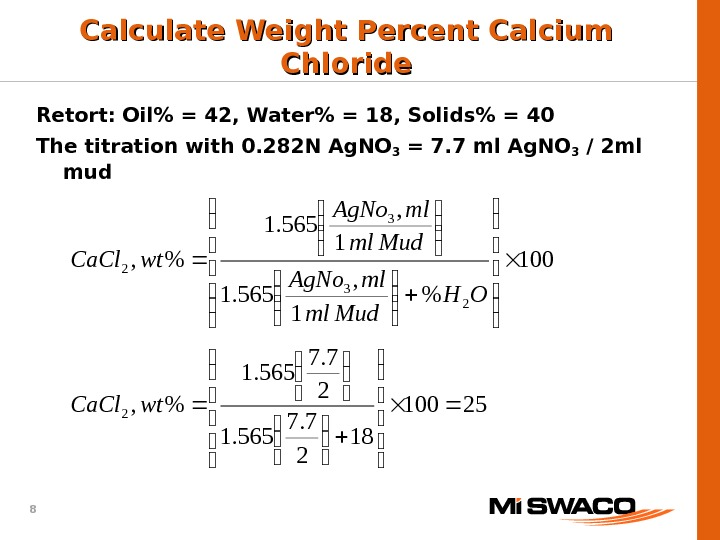8 25100 18 2 7. 7 565. 1 %, 100 % 1 , 565. 1 %, 2 23 3 2 wt. Ca. Cl OH Mudml ml. Ag. No wt. Ca. Cl Calculate Weight Percent Calcium Chloride Retort: Oil% = 42, Water% = 18, Solids% = 40 The titration with 0. 282 N Ag. NO 3 = 7. 7 ml Ag. NO 3 / 2 ml mud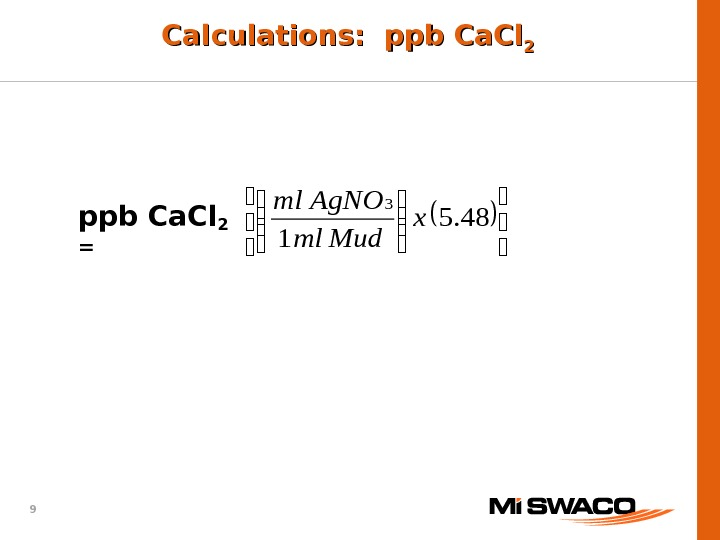9 Calculations: ppb Ca. Cl 22 ppb Ca. Cl 2 = 48. 5 1 3 x Mudml Ag. NOml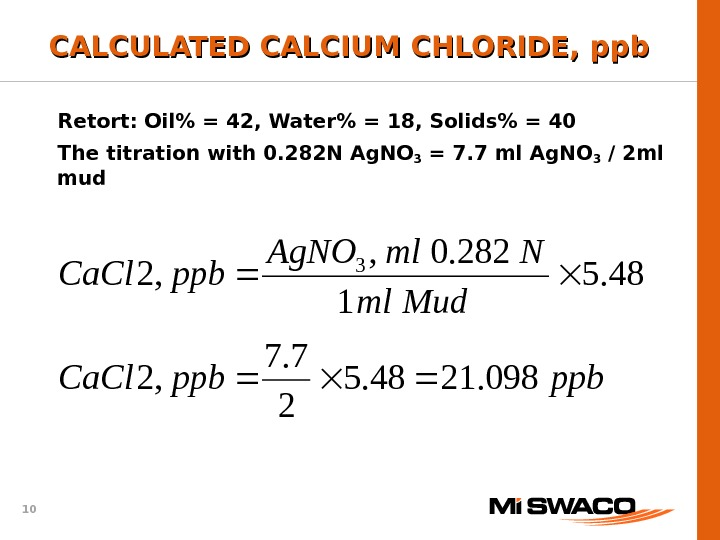10 ppbppb. Ca. Cl Mudml Nml. Ag. NO ppb. Ca. Cl 098. 2148. 5 2 7. 7 , 2 48. 5 1 282. 0, , 2 3 CALCULATED CALCIUM CHLORIDE, ppb Retort: Oil% = 42, Water% = 18, Solids% = 40 The titration with 0. 282 N Ag. NO 3 = 7. 7 ml Ag. NO 3 / 2 ml mud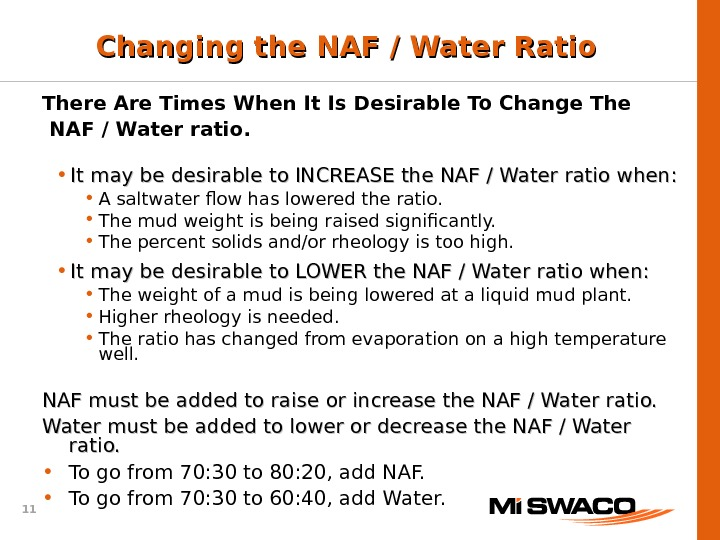11 There Are Times When It Is Desirable To Change The NAF / Water ratio. • It may be desirable to INCREASE the NAF / Water ratio when: • A saltwater flow has lowered the ratio. • The mud weight is being raised significantly. • The percent solids and/or rheology is too high. • It may be desirable to LOWER the NAF / Water ratio when: • The weight of a mud is being lowered at a liquid mud plant. • Higher rheology is needed. • The ratio has changed from evaporation on a high temperature well. NAF must be added to raise or increase the NAF / Water ratio. Water must be added to lower or decrease the NAF / Water ratio. • To go from 70: 30 to 80: 20, add NAF. • To go from 70: 30 to 60: 40, add Water. Changing the NAF / Water Ratio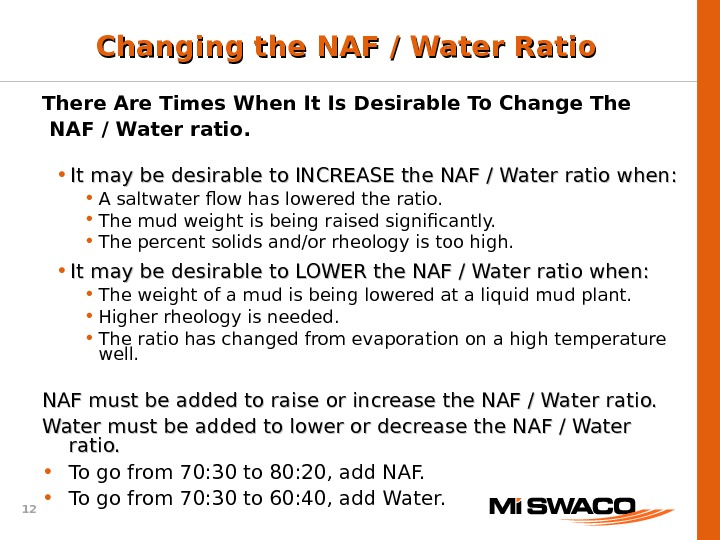12 There Are Times When It Is Desirable To Change The NAF / Water ratio. • It may be desirable to INCREASE the NAF / Water ratio when: • A saltwater flow has lowered the ratio. • The mud weight is being raised significantly. • The percent solids and/or rheology is too high. • It may be desirable to LOWER the NAF / Water ratio when: • The weight of a mud is being lowered at a liquid mud plant. • Higher rheology is needed. • The ratio has changed from evaporation on a high temperature well. NAF must be added to raise or increase the NAF / Water ratio. Water must be added to lower or decrease the NAF / Water ratio. • To go from 70: 30 to 80: 20, add NAF. • To go from 70: 30 to 60: 40, add Water. Changing the NAF / Water Ratio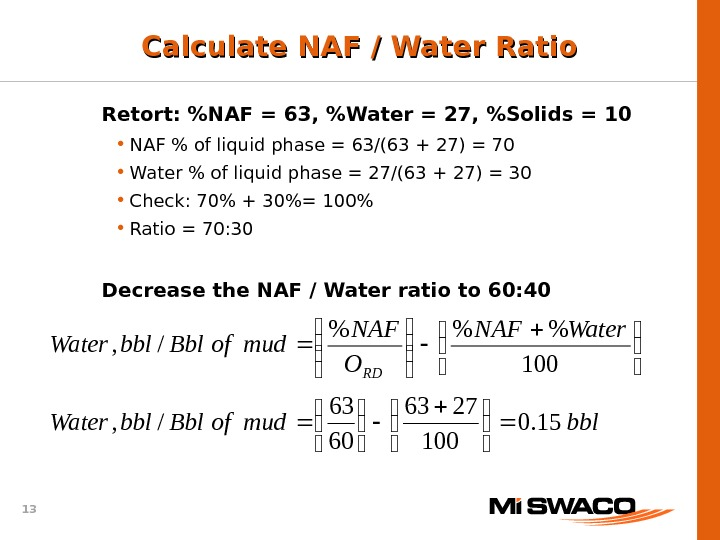13 bblmudof. Bblbbl. Water. NAF O NAF mudof. Bblbbl. Water RD 15. 0 100 2763 60 63 /, 100 %%% /, Calculate NAF / Water Ratio Retort: %NAF = 63, %Water = 27, %Solids = 10 • NAF % of liquid phase = 63/(63 + 27) = 70 • Water % of liquid phase = 27/(63 + 27) = 30 • Check: 70% + 30%= 100% • Ratio = 70: 30 Decrease the NAF / Water ratio to 60: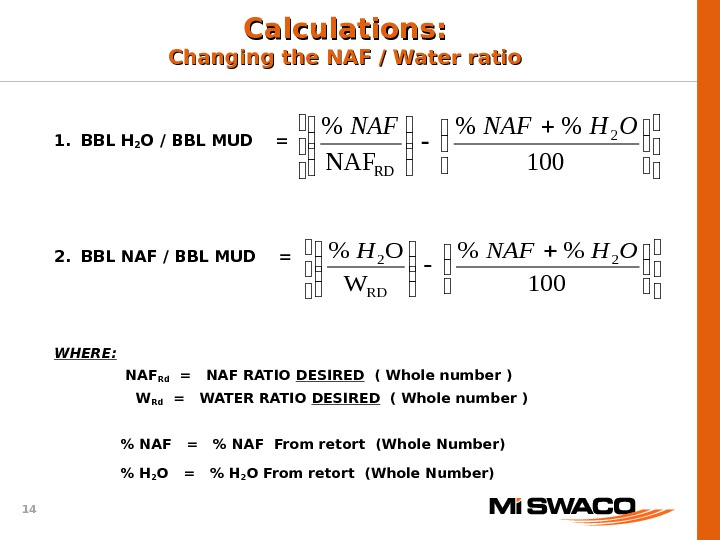14 1. BBL H 2 O / BBL MUD = 2. BBL NAF / BBL MUD = WHERE: NAF Rd = NAF RATIO DESIRED ( Whole number ) W Rd = WATER RATIO DESIRED ( Whole number ) % NAF = % NAF From retort (Whole Number) % H 2 O = % H 2 O From retort (Whole Number)Calculations: Changing the NAF / Water ratio 100 %% W O%2 RD 2 OHNAFH 100 %% NAF% 2 RD OHN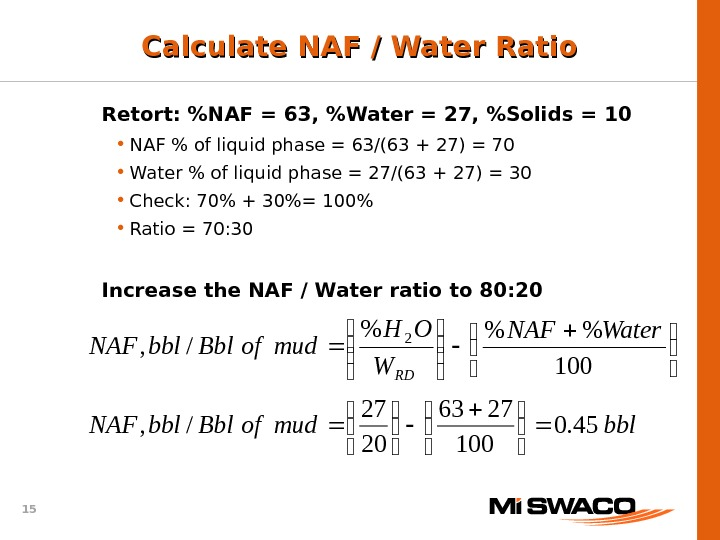15 bblmudof. Bblbbl. NAF Water. NAF W OH mudof. Bblbbl. NAF RD 45. 0 100 2763 20 27 /, 100 %%% /, 2 Calculate NAF / Water Ratio Retort: %NAF = 63, %Water = 27, %Solids = 10 • NAF % of liquid phase = 63/(63 + 27) = 70 • Water % of liquid phase = 27/(63 + 27) = 30 • Check: 70% + 30%= 100% • Ratio = 70: 30 Increase the NAF / Water ratio to 80: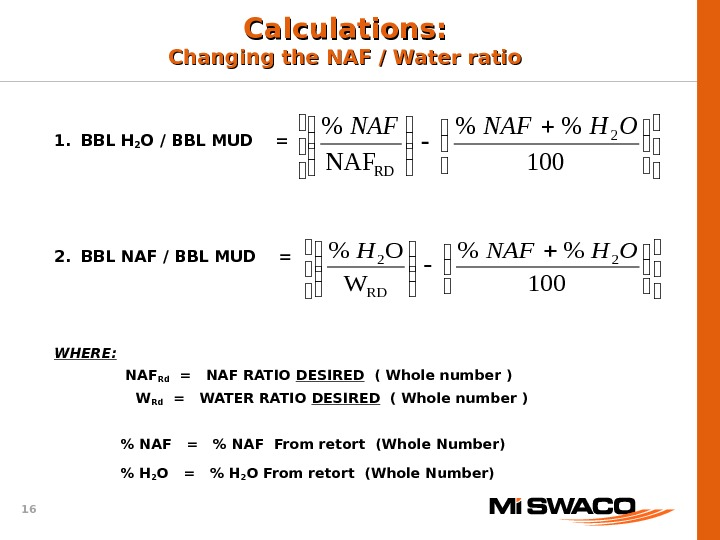16 1. BBL H 2 O / BBL MUD = 2. BBL NAF / BBL MUD = WHERE: NAF Rd = NAF RATIO DESIRED ( Whole number ) W Rd = WATER RATIO DESIRED ( Whole number ) % NAF = % NAF From retort (Whole Number) % H 2 O = % H 2 O From retort (Whole Number)Calculations: Changing the NAF / Water ratio 100 %% W O%2 RD 2 OHNAFH 100 %% NAF% 2 RD OHN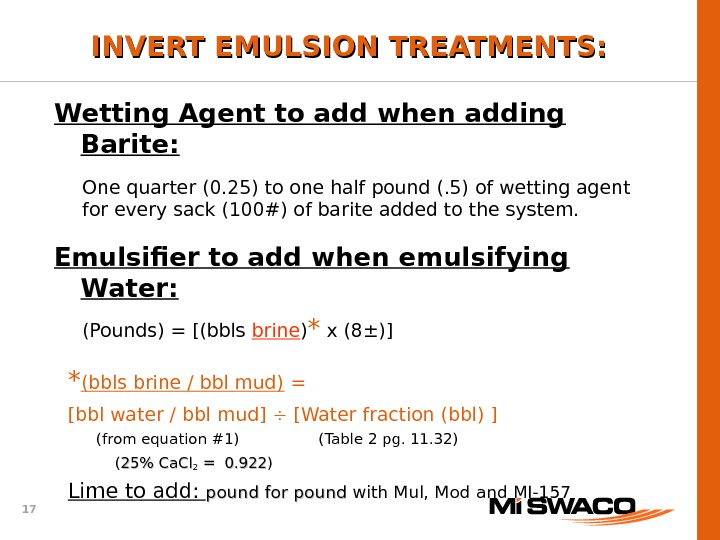17 Wetting Agent to add when adding Barite: One quarter (0. 25) to one half pound (. 5) of wetting agent for every sack (100#) of barite added to the system. Emulsifier to add when emulsifying Water: (Pounds) = [(bbls brine ) * x (8 ± )] * (bbls brine / bbl mud) = [bbl water / bbl mud] [Water fraction (bbl) ] (from equation #1) (Table 2 pg. 11. 32) ( 25% Ca. Cl 22 = 0. 922 ) Lime to add: pound for pound with Mul, Mod and MI-157 INVERT EMULSION TREATMENTS: